###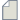Author Topic: How to know how much time my simulation will take before running?  (Read 372 times)

0 Members and 1 Guest are viewing this topic.

####Kevin Joven

• New ATK user
•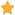• Posts: 1
• Country: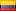• Reputation: 0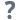##### How to know how much time my simulation will take before running?
« on: December 14, 2021, 09:23 »
Dear community,
I am new in QuantumATK running some particular applications. Some of them take so much time. I would like to know how much time my simulation will take before running or if there is some method to estimate that time (based on some parameters).

####filipr

• QuantumATK Staff
• Regular ATK user
•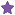• Posts: 28
• Country:• Reputation: 3
• QuantumATK developer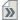##### Re: How to know how much time my simulation will take before running?
« Reply #1 on: December 15, 2021, 13:25 »
Hi Kevin,

In general you can't know how long time a calculation will take. First of all, it depends on what kind of calculation you are doing: Molecular Dynamics with Force Fields? A transmission spectrum calculation using a tight-binding calculator? Or a band structure calculation using DFT? Many calculations involve multiple steps, e.g. a DFT band structure first requires you to determine the self-consistent ground state density, after which you can calculate the band structure.

In some cases you can estimate the order of magnitude, but let's consider an example to give you an idea of the complexity involved:

Consider a DFT calculation: It is an iterative approach: Given an effective potential you calculate the density, then up update the potential, calculate a new density and so on until the change in the density between subsequent steps is smaller than some threshold. How many steps will this take? There is no way of knowing, as it depends on the system, pseudopotentials, numerical settings etc - but it is typically between 10 and 100 steps. Now in each step you calculate the Hamiltonian and find it's eigenvalues. The Hamiltonian has several contributions: For instance calculating the XC potential scales as the number of grid points, i.e. with the volume, solving the electrostatic potential in general scales as the number of grid points squared. Finding the eigenvalues and eigenvectors of the Hamiltonian scales cubicly in the basis set size, which itself is proportional to the volume or equivalently the number of atoms. Due to the prefactors of the different terms the calculation will be limited by different calculations for different systems. If you have a small to medium system with a high grid point density it may be limited by grid terms like XC and electrostatic potentials, whereas for large systems the cubic scaling of the basis set size will surely dominate. Estimating the time for each contribution from to the total calculation is extremely hard and depends on settings, parallelization and the computer specs.

The best you can do is to make different sized versions of the system you want to study, e.g. a smallish version, a medium version and a large version, run them and then extrapolate the timings assuming the N³ scaling behavior for large systems. Note this assumes that each version uses the same number of SCF steps... You may want to only take the time per SCF step into account.

Now this was a single DFT calculation. What if you also want to do geometry optimization/relaxation? Well, such a calculation is also an iterative algorithm that may take between 1 and infinitely many steps at each step doing a DFT calculation.

All of this timing analysis has to be repeated for every type of calculation, parameters, parallelization etc etc etc.

So in practice you don't estimate the time. You can make a couple of "sounding" calculations, i.e. smaller and faster calculations that consider a smaller part of the full system you want to describe, to get an idea of convergence, precision and timing. Then from those you can guesstimate or extrapolate the approximate time scale needed for the full scale model. When you have done a couple (or many!) calculations you start to get a vague idea of the time it takes to do similar calculations.
« Last Edit: December 15, 2021, 13:30 by filipr »